# What is the theorem of 30 60 90

What is a 30-60-90 triangle?

 ...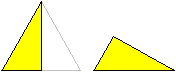... If you halve an equilateral triangle by a height, you get a triangle with interior angles of 30 °, 60 ° and 90 °.That is why it is also called the 30-60-90 triangle.
The special thing about this triangle is that the interior angles are in a ratio of 1: 2: 3.

When a triangle is mentioned on this page, it is usually the 30-60-90 triangle.

Sizes of the triangle Top

 ...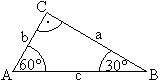... If the hypotenuse of the exit triangle is the same cso are the cathets b= (1/2) c and a= (1/2) sqr (3) c. The following applies to the aspect ratio:b:c = sqrt (3):1:2.The area is A.= (1/2) ab = (1/8) sqr (3) c².
The scope is U= a + b + c = (1/2) [(3 + sqr (3)] c.

 ...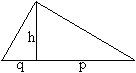... Since the 30-60-90 triangle is right-angled, there is only one height h, the other heights coincide with the cathetus. The height divides the hypotenuse c into the hypotenuse sections p and q.
It applies H= (1/4) sqr (3) c [follows from the area formulas (1/2) ab = (1/2) ch]
and p= (3/4) c, q= (1/4) c [follows from the cathetus set pc = b² or qc = a²].

 ...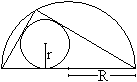... Like every triangle, the 30-60-90 triangle has a perimeter and an inscribed circle.Half of the circumference is the circle of the valley with the radius R.= c / 2.The radius of the inscribed circle is r= (1/4) [3 - sqr (3)] c.
 ...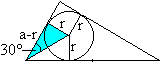... Look at the blue triangle. The following applies: tan (30 °) = r / (a-r). If one sets tan (30 °) = 1 / sqr (3) and a = c / 2, after some transformations r = (1/4) [3 - sqr (3)] c results.

Squares in the triangle Top

 ...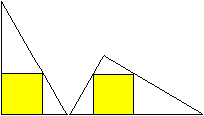... There are two ways to put a square inside a 30-60-90 triangle.Are the squares the same size?

solution
 ...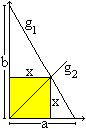... To solve this, the triangle is placed in a coordinate system and the straight line g is considered1 and G2. The straight line g1 : f (x) = - (b / a) * x + b contains the hypotenuse,the straight line g2 : g (x) = x is the 1st bisector and contains two corners of the square.For the intersection of the two straight lines, f (x) = g (x) or x = -b / a * x + b or applies x = ab / (a ​​+ b).
Derivation
x = -b / a * x + b | * a
ax = -bx + ab | + bx
ax + bx = ab | Exclude x
(a + b) x = ab |: (a + b)
x = ab / (a ​​+ b)
 ...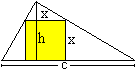... According to the 2nd theorem of rays (slightly modified), h: c = (h-x): x applies. It follows x = hc / (h + c).
The results are remarkable: the half-square sides are a harmonious mean in both cases, on the one hand that of height and hypotenuse, on the other hand that of the two cathets.
Incidentally, the statements apply to any right-angled triangles.

If you insert the sizes of the 30-60-90 triangle, the result is
in the first case x = (1/4) [3-sqr (3)] c (about 0.32c),
in the second case x = (1/13) [4sqr (3) -3] c (about 0.30c).

Figures made from 30-60-90 triangles Top
Squares, equilateral triangles or isosceles right-angled triangles can be put together in such a way that new figures are created. They are then called polyominos (pentominos or hexominos), Polyiamonds or polybolos.
Of course, you can also create new figures from 30-60-90 triangles.

 ...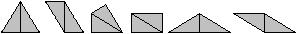... You can put two triangles together in six different ways. They are not well suited as puzzle pieces because all routes are different from each other.
The question is: How many figures can you lay out of three (four, ...) triangles?
However: These figures appear in the Eternity game and are called Polydrafter (see link list).

Figures made up of six triangles
 ...... If you draw all heights in an equilateral triangle, you get six 30-60-90 triangles. You can use them as tangram stones. (1)

A figure made up of eight triangles
 ...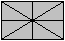... If you draw the diagonals and the center lines in a rectangle, you get eight right-angled triangles.The figure corresponds to the English national flag. In general, the triangles are not 30-60-90 triangles. This only applies if the flag has the format sqr (3): 1.
For comparison: The Din format has the aspect ratio sqr (2): 1.

spiral
 ...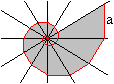... Six straight lines meet at one point and form 12 angles of size 30 °.If one specifies the vertical line of length a and continues it in the angular spaces by drawing a perpendicular to the next half-line, a sequence of ever smaller 30-60-90 triangles is created. The shorter cathets form a spiral (red).What limit does the length of the spiral approach if a is given?

solution
 ...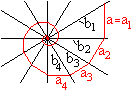... Given is a.The sequence of hypotenuses b1, b2, b3, b4, ... form a geometric sequence.
b1= 2a
b2 = [(1/2) sqr (3)] (2a)
b3 = [(1/2) sqr (3)] b2 = [(1/2) sqr (3)] ² (2a)
b4 = [(1/2) sqr (3)] b3 = [(1/2) sqr (3)] ³ (2a)
...

The following applies to the shorter catheters that form the spiral:
a1 = a
a2 = (1/2) b2 = [(1/2) sqr (3)] a
a3 = (1/2) b3 = [(1/2) sqr (3)] ²a
a4 = (1/2) b4 = [(1/2) sqr (3)] 3 a
...
That is a geometric sequence. The associated series has the limit value 1 / (1-q) = [4 + 2sqr (3)] a

Two more figures
 ...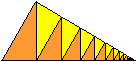...Triangles in a 30-60-90 triangle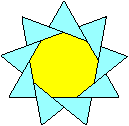A star made up of nine triangles

Two cones Top

 ...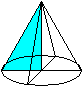...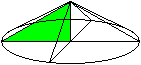... Two cones are created when the triangle rotates around one of the two cathets.Let V (a) be the volume when rotating around a, V (b) around b.The volumes of the two cones are in the ratio V (b): V (a) = sqr (3): 1.

The triangle can also rotate around the hypotenuse. Then a double cone arises with V (q): V (p) = 3: 1.

Eternity puzzle Top

 ...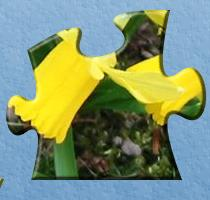... ...... Puzzles are known in which one should put together a rectangular picture from individual pieces.In this case there are 24 pieces.

In June 1999 the British company Racing Champions Ltd brought the so-called Eternity Puzzle onto the market. The inventor was Christopher Monckton. According to (2) the puzzle has been sold more than 250,000 times.

 ...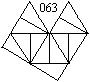... ...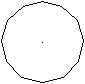... The puzzle consists of 209 pieces. Each part is made up of twelve 30-60-90 triangles.With these parts an almost regular dodecagon should be laid out.If a is the length of the side and h is the height of the equilateral triangle, the dodecagon has alternating sides 7a and 8h.
In May 2000, two mathematicians from Cambridge solved the puzzle first - with the help of a computer.
You can find more via my link list.

30-60-90 triangle on the internet Top

German

Wikipedia
Eternity puzzle

English

Eric W. Weisstein (MathWorld)
30-60-90 Triangle, Eternity, Polydrafter

Ed Pegg Jr. (Math Puzzles)
THE ETERNITY PUZZLE

John Page
30 ° - 60 ° - 90 ° triangle

Lawrence Spector (TheMathPage)
THE 30 ° -60 ° -90 ° TRIANGLE

Wikipedia
30-60-90 triangle, Eternity puzzle

credentials Top
(1) Karl-Heinz Koch: ... lege Spiele, Cologne 1987 (ISBN 3-7701-2097-3)
(2) Ingo Althöfer: One million British pounds for two mathematicians, Omega magazine, Spektrum Spezial 4/2003

Feedback: Email address on my main page

URL of my homepage:
http://www.mathematische-basteleien.de/